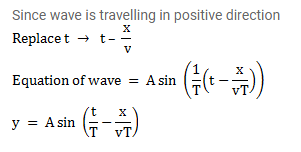# The displacement of the particle at x=0 of a stretched string carrying`
Question:

The displacement of the particle at $x=0$ of a stretched string carrying a wave in the positive $x$-direction is given by $\mathrm{f}(\mathrm{t})=\mathrm{A} \sin (\mathrm{t} / \mathrm{T})$

The wave speed is $v$. Write the wave equation.

Solution: